Question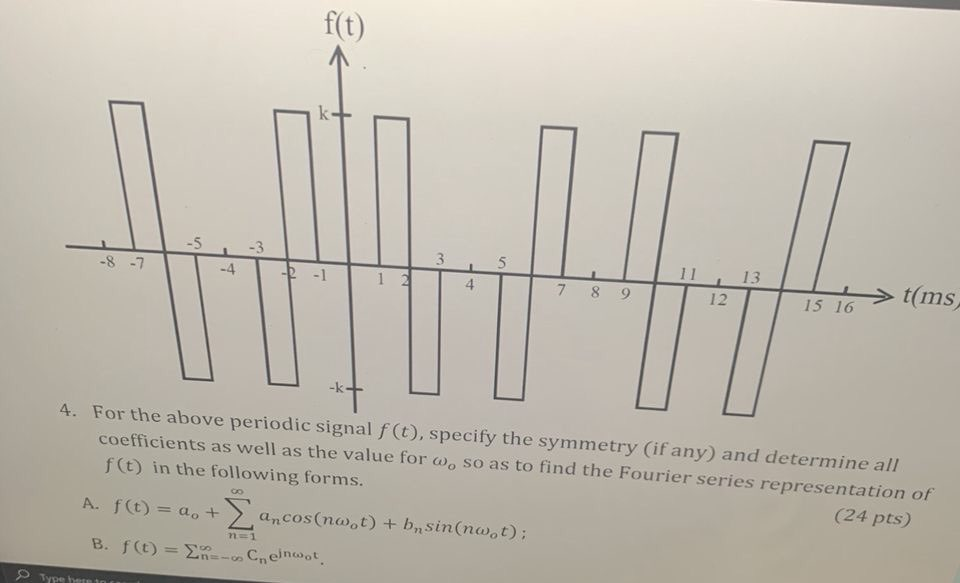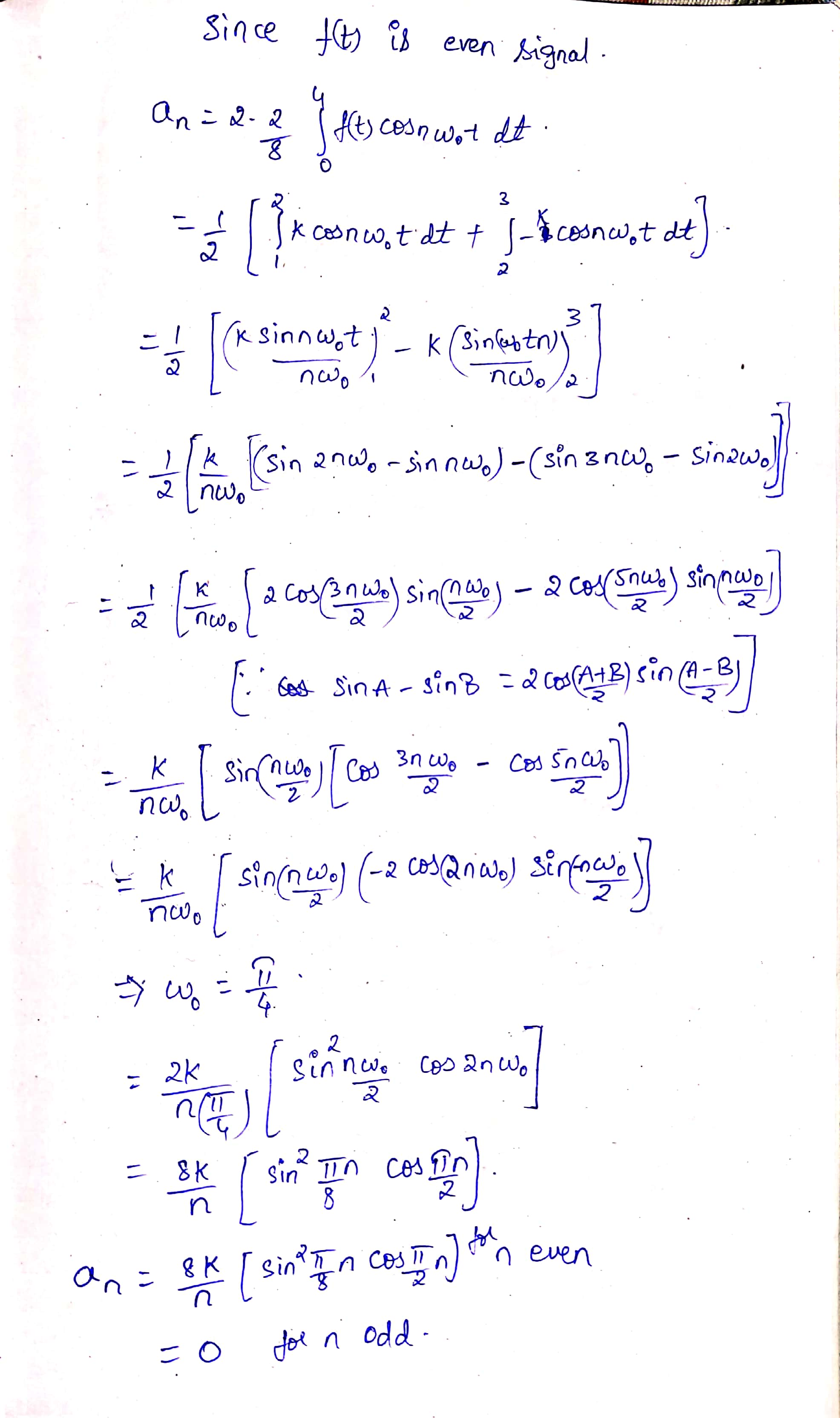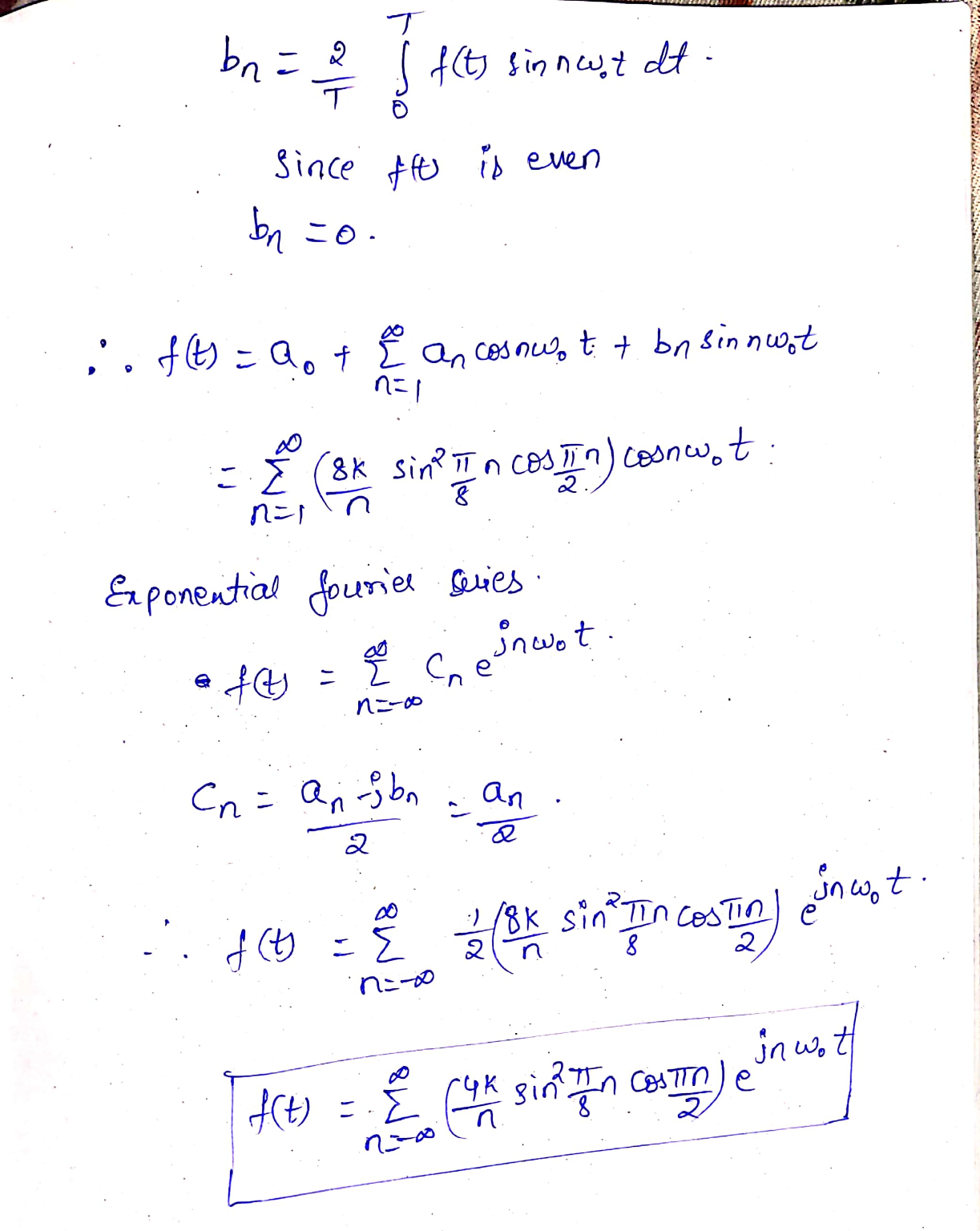#### Earn Coins

Coins can be redeemed for fabulous gifts.

Similar Homework Help Questions
• ### Problem 1 (20 pts) Suppose that x(t) = e 2 for 0 st <3 and is...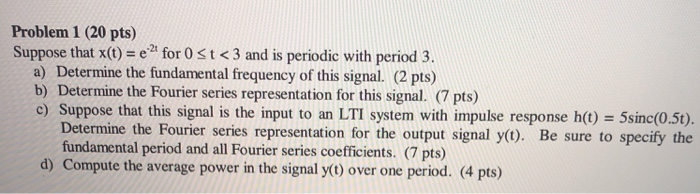Problem 1 (20 pts) Suppose that x(t) = e 2 for 0 st <3 and is periodic with period 3. a) Determine the fundamental frequency of this signal. (2 pts) b) Determine the Fourier series representation for this signal. (7 pts) c) Suppose that this signal is the input to an LTI system with impulse response h(t) = 5sinc(0.5t). Determine the Fourier series representation for the output signal y(t). Be sure to specify the fundamental period and all Fourier series...

• ### Consider the following CT periodic signals x(t), y(t) and z(t) a(t) 5 -4 y(t) 5/-4 z(t)...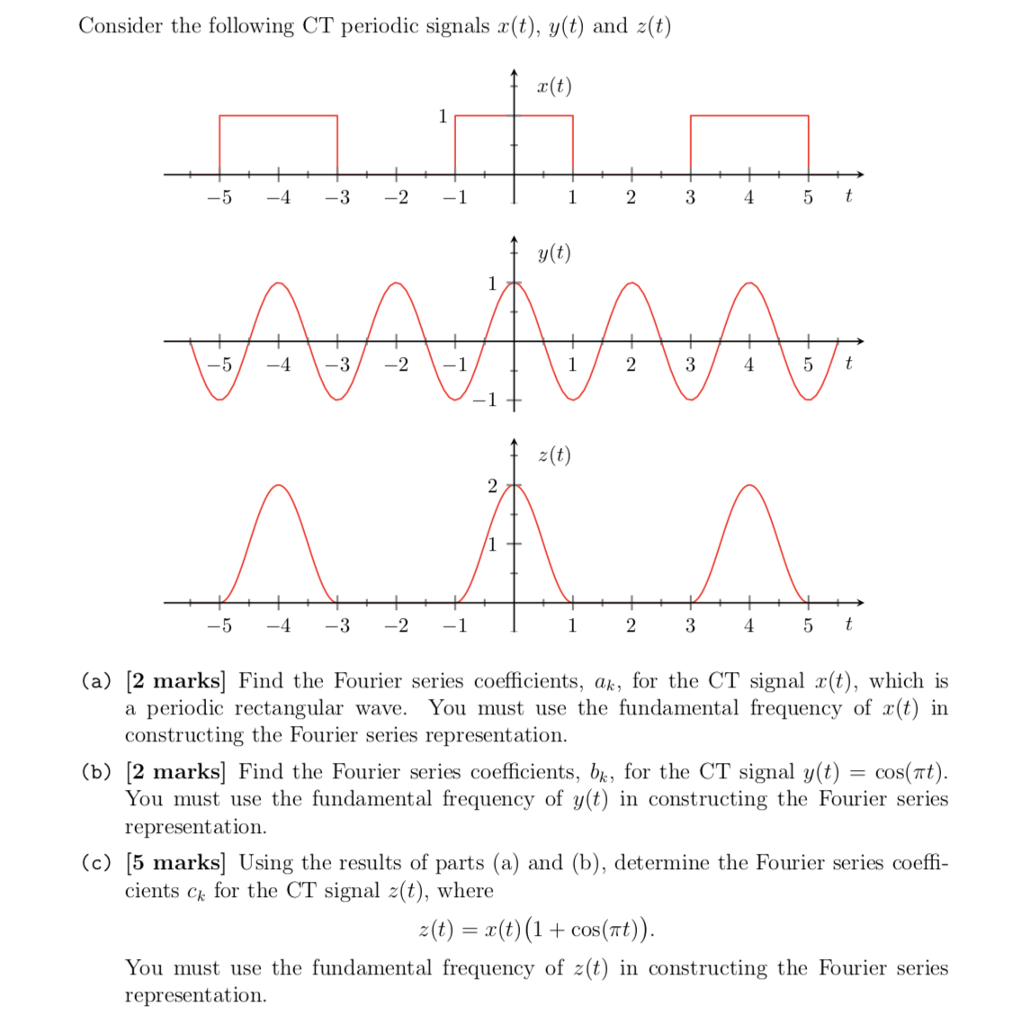Consider the following CT periodic signals x(t), y(t) and z(t) a(t) 5 -4 y(t) 5/-4 z(t) 5 4 (a) [2 marks] Find the Fourier series coefficients, ak, for the CT signal r(t), which is a periodic rectangular wave. You must use the fundamental frequency of r(t) in constructing the Fourier series representation (b) [2 marks] Find the Fourier series coefficients, bk, for the CT signal y(t) cos(t) You must use the fundamental frequency of y(t) in constructing the Fourier series...

• ### (b) Let X(ju) denote the Fourier transform of the signal r(t) shown in the figure x(t) 2 -2 1 2 Using the properties of the Fourier transform (and without explicitly evaluating X(jw)), ii. (5 pts)...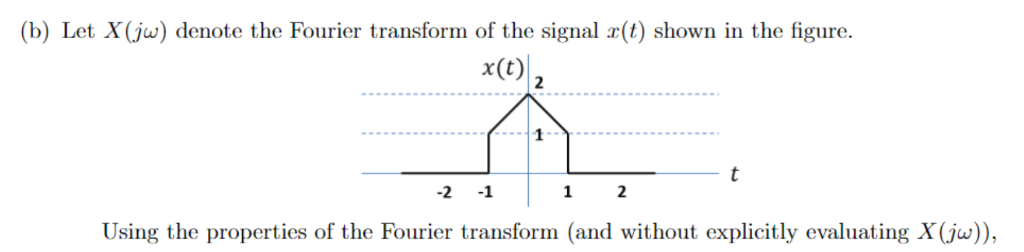(b) Let X(ju) denote the Fourier transform of the signal r(t) shown in the figure x(t) 2 -2 1 2 Using the properties of the Fourier transform (and without explicitly evaluating X(jw)), ii. (5 pts) Find2X(jw)dw. Hint: Apply the definition of the inverse Fourier transform formula, and you can also recall the time shift property for Fourier Transform. (c) (5 pts) Fourier Series. Consider the periodic signal r(t) below: 1 x(t) 1 -2 ·1/4 Transform r(t) into its Fourier Series...

• ### Problem 32: (20 points) Consider a periodic signal f(t), with fundamental period To, that has the...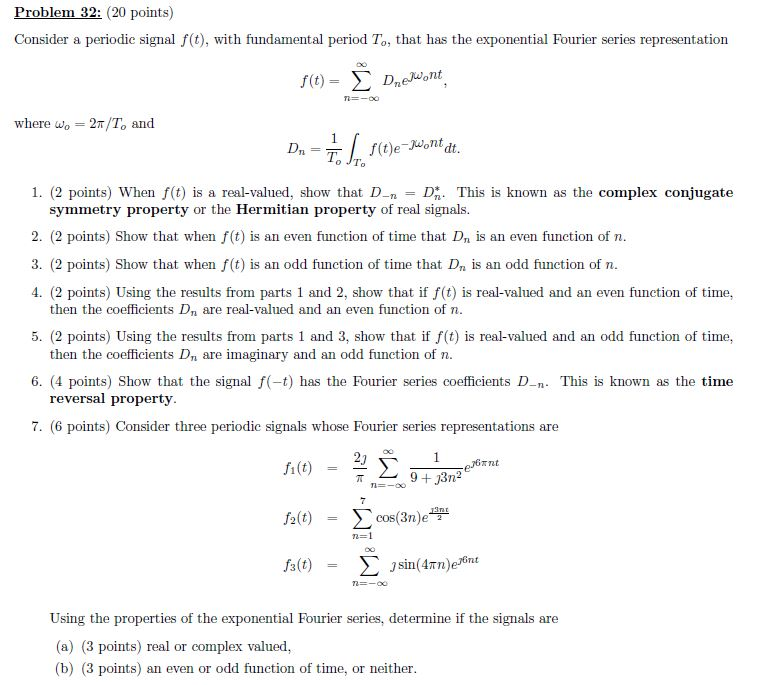Problem 32: (20 points) Consider a periodic signal f(t), with fundamental period To, that has the exponential Fourier series representation f(t) = Σ Dnejuont . where wo 2T/To and 1. (2 points) When f(t) is a real-valued, show that DD This is known as the complex conjugate symmetry property or the Hermitian property of real signals. 2. (2 points) Show that when f(t) is an even function of time that Dn is an even function of n 3. (2 points)...

• ### A periodic signal f(t) is produced by periodically repeating the function alt) - S2t|t| for -1<t<1...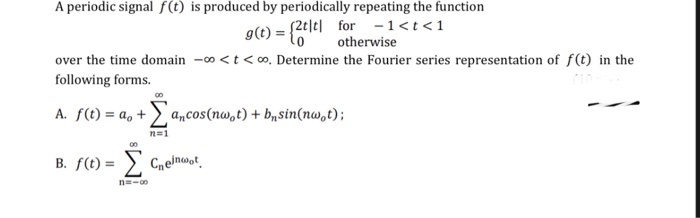A periodic signal f(t) is produced by periodically repeating the function alt) - S2t|t| for -1<t<1 g(t) = to otherwise over the time domain-00<t<0. Determine the Fourier series representation of f(t) in the following forms. A. f(t) = a, + acos(nw,t) + b sin(nw,t); na1 B. f(0) = { Chelmuese n -00

• ### 5. (12 points) Consider a continuous-time LTI system whose frequency response is sin(w) H(ju) 4w If...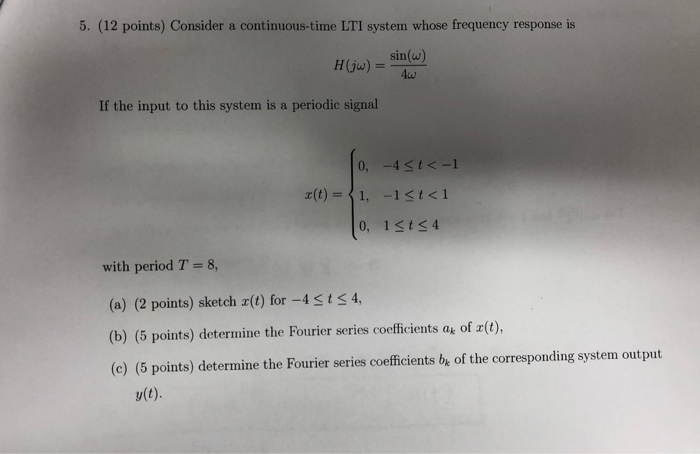5. (12 points) Consider a continuous-time LTI system whose frequency response is sin(w) H(ju) 4w If the input to this system is a periodic signal 0, -4<t<-1 x(t)=1, -1st<1 0, 1st<4 with period T= 8 (a) (2 points) sketch r(t) for -4ts4 (b) (5 points) determine the Fourier series coefficients at of x(t), (c) (5 points) determine the Fourier series coefficients be of the corresponding system output y(t) 5. (12 points) Consider a continuous-time LTI system whose frequency response is...

• ### 3) (Symmetries and Fourier Coefficients) Compute the Fourier Series Coefficients a, b and XTk] for the following periodic repeating signals. Where appropriate, simplify the results for odd or even va...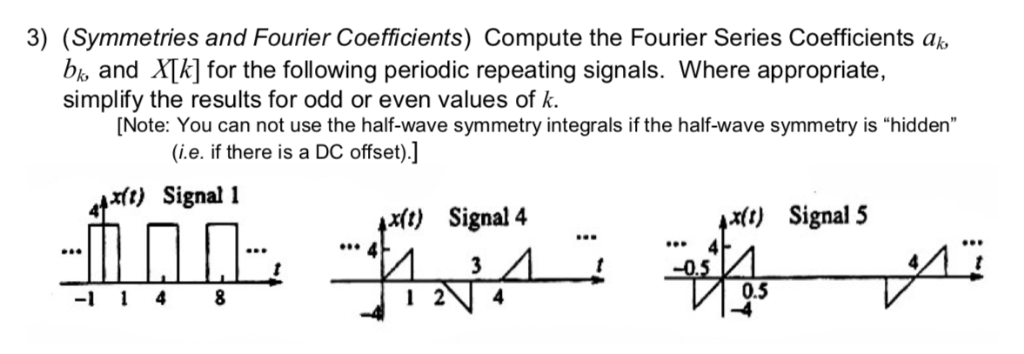3) (Symmetries and Fourier Coefficients) Compute the Fourier Series Coefficients a, b and XTk] for the following periodic repeating signals. Where appropriate, simplify the results for odd or even values of k. Note: You can not use the half-wave symmetry integrals if the half-wave symmetry is "hidden" (i.e. if there is a DC offset).] xft) Signal i x(t) Signal5 x(t) Signal 4 aeP O80 0.5 -1 4 8 I 2 4 3) (Symmetries and Fourier Coefficients) Compute the Fourier Series...

• ### 1. Using the Fourier series analysis Equation 3 for the periodic function r(t) shown in Figure 2....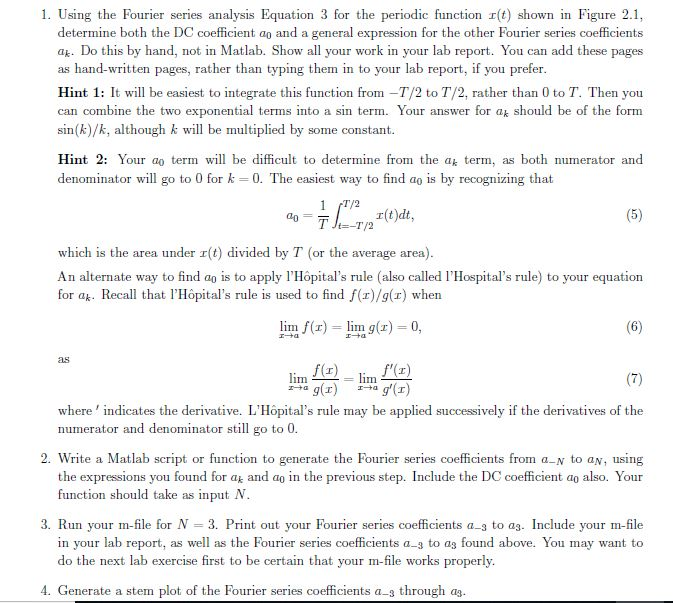1. Using the Fourier series analysis Equation 3 for the periodic function r(t) shown in Figure 2.1, determine both the DC coefficient ao and a general expression for the other Fourier series coefficients ak. Do this by hand, not in Matlab. Show all your work in your lab report. You can add these pages as hand-written pages, rather than typing them in to your lab report, if you prefer Hint 1: It will be easiest to integrate this function from...

• ### 4. Consider the Fourier series for the periodic function given below: x(t) = 3 + 5Cost...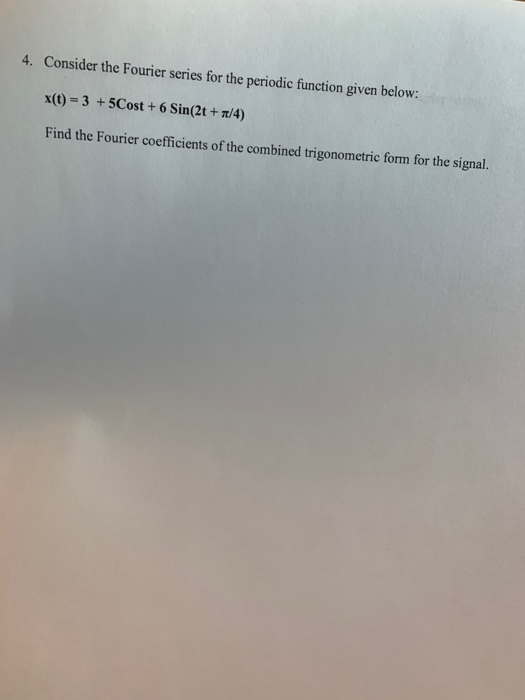4. Consider the Fourier series for the periodic function given below: x(t) = 3 + 5Cost + 6 Sin(2t + /4) Find the Fourier coefficients of the combined trigonometric form for the signal.

• ### efficients Wi for the signal w(t) sin(16rt) 14. (8 pts) Determine the FS coefficients W, for the signal w(t) 3 cos(12rt)-5 sin(16rt). (c) (9 pts) Suppose a MATLAB anonymous function X already...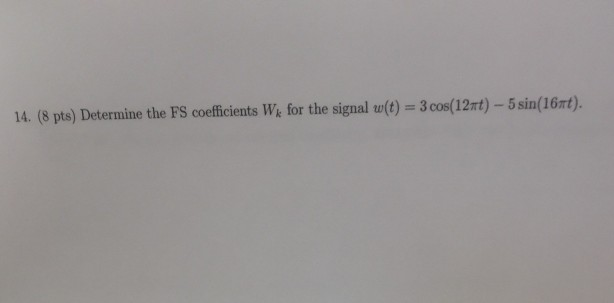efficients Wi for the signal w(t) sin(16rt) 14. (8 pts) Determine the FS coefficients W, for the signal w(t) 3 cos(12rt)-5 sin(16rt). (c) (9 pts) Suppose a MATLAB anonymous function X already exists that correctly defines X() Write MATLAB code that uses X to reconstruct and then plot a 10-periodic replication of e(t) over-10sts10. efficients Wi for the signal w(t) sin(16rt) 14. (8 pts) Determine the FS coefficients W, for the signal w(t) 3 cos(12rt)-5 sin(16rt). (c) (9 pts) Suppose...